# Aswath Damodaran SESSION 4 EQUITY RISK PREMIUMS DCF

• Slides: 14Aswath Damodaran SESSION 4: EQUITY RISK PREMIUMS DCF Valuation 1The Price of Equity Risk 2 Aswath Damodaran 2Equity Risk Premiums: Intuition 3 The equity risk premium is the premium that investors charge for investing in the average equity. It is a function of � How risk averse investors are collectively � How much risk they see in the average equity The level of the equity risk premium should vary over time as a function of: � Changing macro economic risk (inflation & GDP growth) � The fear of catastrophic risk � The transparency in financial statements and governance Aswath Damodaran 3Equity Risk Premiums The ubiquitous historical risk premium 4 Aswath Damodaran 4The perils of trusting the past……. 5 The estimates are noisy. Even with 80 years of data, the standard error in US stocks is 2. 26%. Standard Error in Premium = 20%/√ 80 = 2. 26% Using historical data from the U. S. equity markets over the twentieth century creates a sampling bias. Aswath Damodaran 5A Forward Looking, Dynamic ERP Aswath Damodaran 6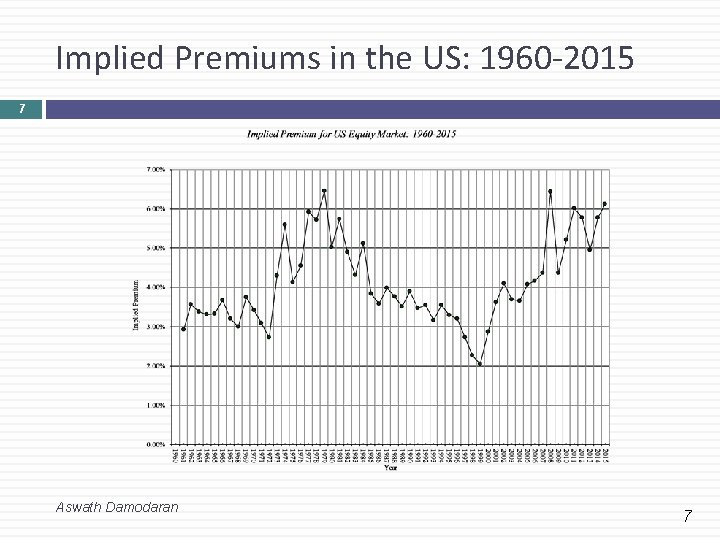Implied Premiums in the US: 1960 -2015 7 Aswath Damodaran 7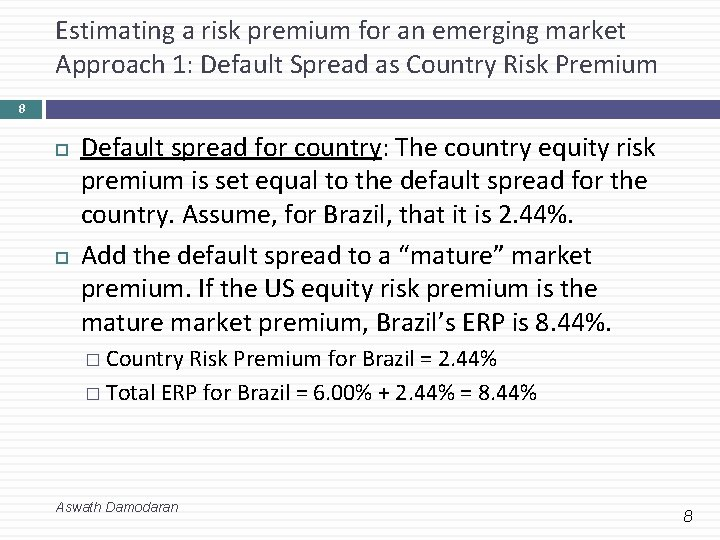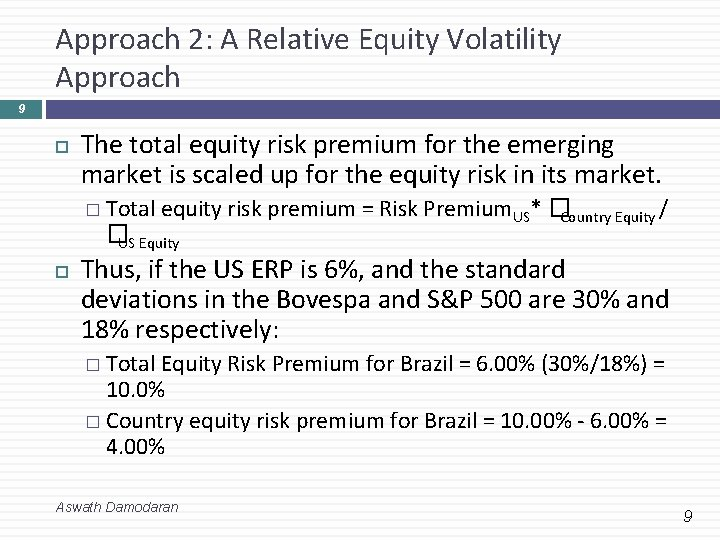Approach 2: A Relative Equity Volatility Approach 9 The total equity risk premium for the emerging market is scaled up for the equity risk in its market. � Total equity risk premium = Risk Premium. US* �Country Equity / �US Equity Thus, if the US ERP is 6%, and the standard deviations in the Bovespa and S&P 500 are 30% and 18% respectively: � Total Equity Risk Premium for Brazil = 6. 00% (30%/18%) = 10. 0% � Country equity risk premium for Brazil = 10. 00% - 6. 00% = 4. 00% Aswath Damodaran 9Approach 3: A melded approach to estimating the additional country risk premium 10 Country ratings measure default risk. Equity is riskier than the government bond. Scale up default spread by the relative volatility of stock and bond prices in that market. Using this approach for Brazil in January 2016, you would get: Standard Deviation in Bovespa (Equity) = 30% Standard Deviation in Brazil government bond = 20% Default spread for Brazil= 2. 44% � Brazil Country Risk Premium = 2. 44% (30%/20%) = 3. 66% � Brazil Total ERP = Mature Market Premium + CRP = 6. 00% + 3. 66% = 9. 66% Aswath Damodaran 10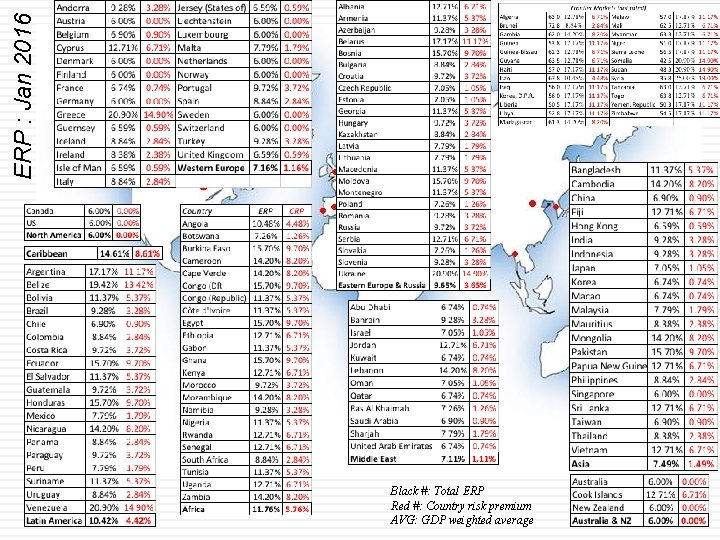ERP : Jan 2016 Black #: Total ERP Red #: Country risk premium AVG: GDP weighted average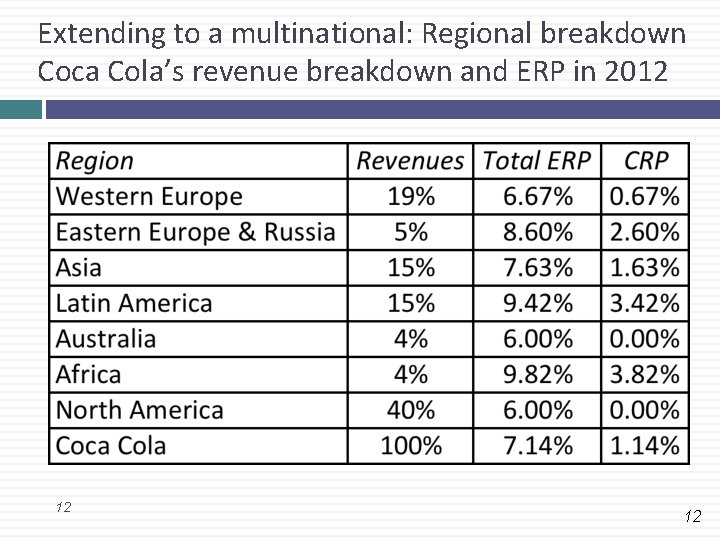Extending to a multinational: Regional breakdown Coca Cola’s revenue breakdown and ERP in 2012 12 12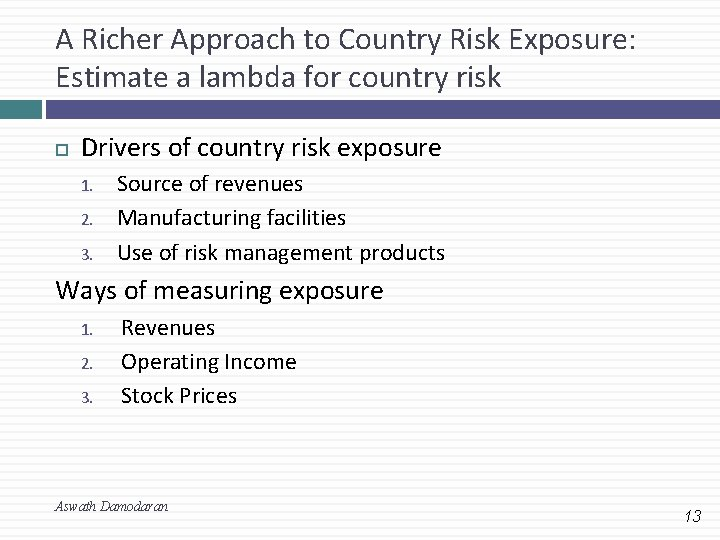A Richer Approach to Country Risk Exposure: Estimate a lambda for country risk Drivers of country risk exposure 1. 2. 3. Source of revenues Manufacturing facilities Use of risk management products Ways of measuring exposure 1. 2. 3. Revenues Operating Income Stock Prices Aswath Damodaran 13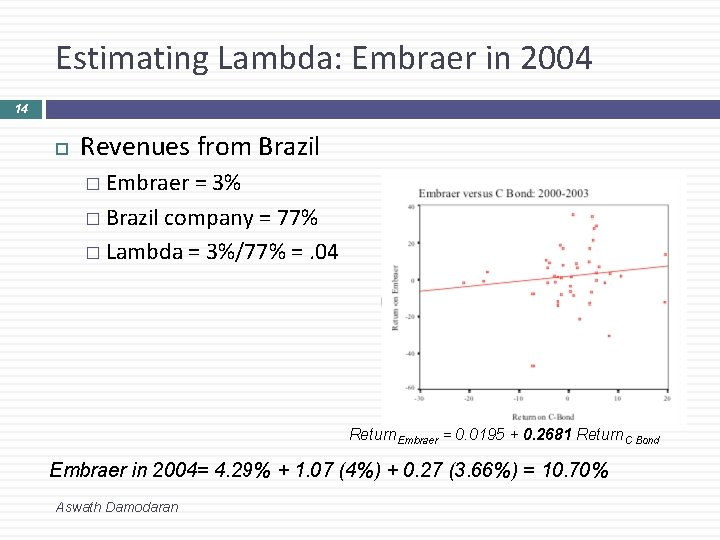Estimating Lambda: Embraer in 2004 14 Revenues from Brazil � Embraer = 3% � Brazil company = 77% � Lambda = 3%/77% =. 04 Return. Embraer = 0. 0195 + 0. 2681 Return. C Bond Embraer in 2004= 4. 29% + 1. 07 (4%) + 0. 27 (3. 66%) = 10. 70% Aswath Damodaran